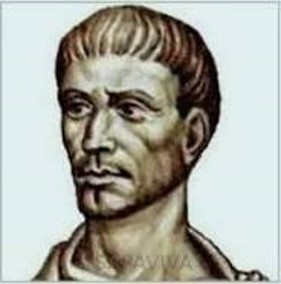## DiophantusDiophantus of Alexandria was an Alexandrian Hellenistic mathematician, who was the author of a series of books called Arithmetica, many of which are now lost. His texts deal with solving algebraic equations. The study of Diophantine equations (“Diophantine geometry”) and of Diophantine approximations remain important areas of mathematical research.

Diophantus made important advances in mathematical notation, becoming the first person known to use algebraic notation and symbolism. Before him everyone wrote out equations in words. He introduced an algebraic symbolism that used an abridged notation for frequently occurring operations, and an abbreviation for the unknown and for the powers of the unknown.